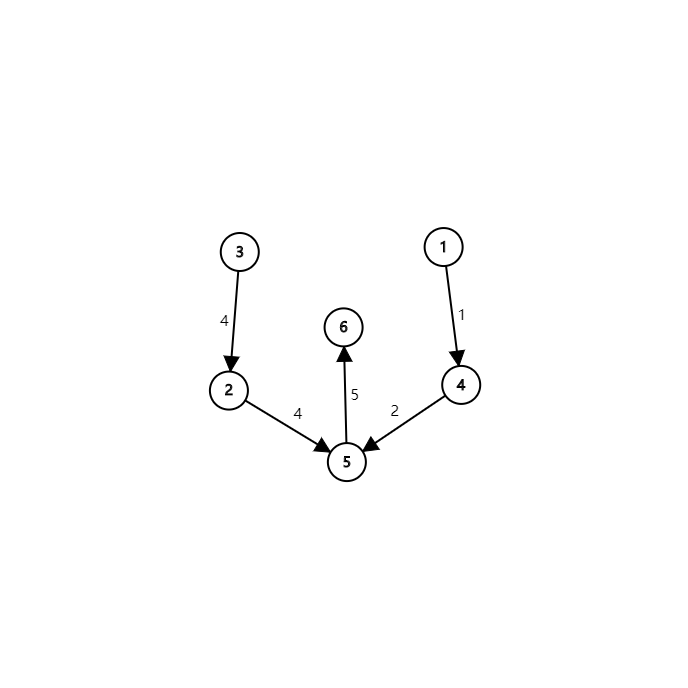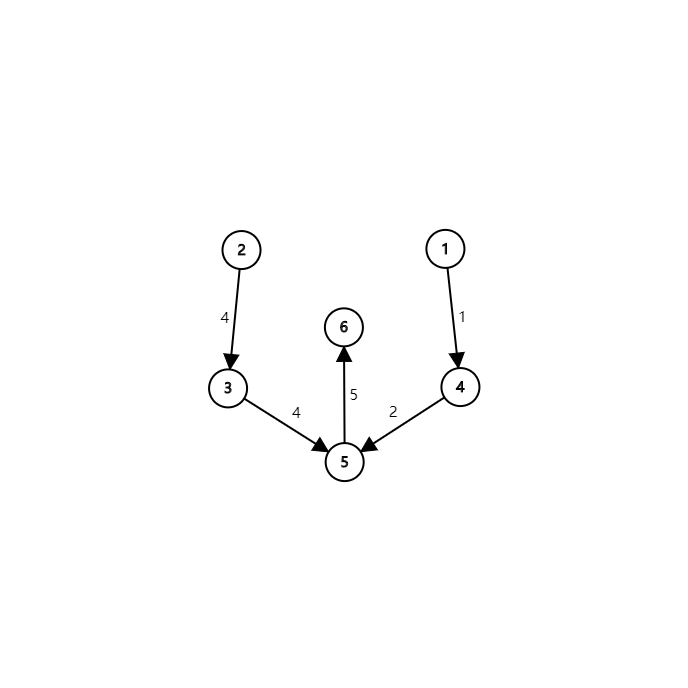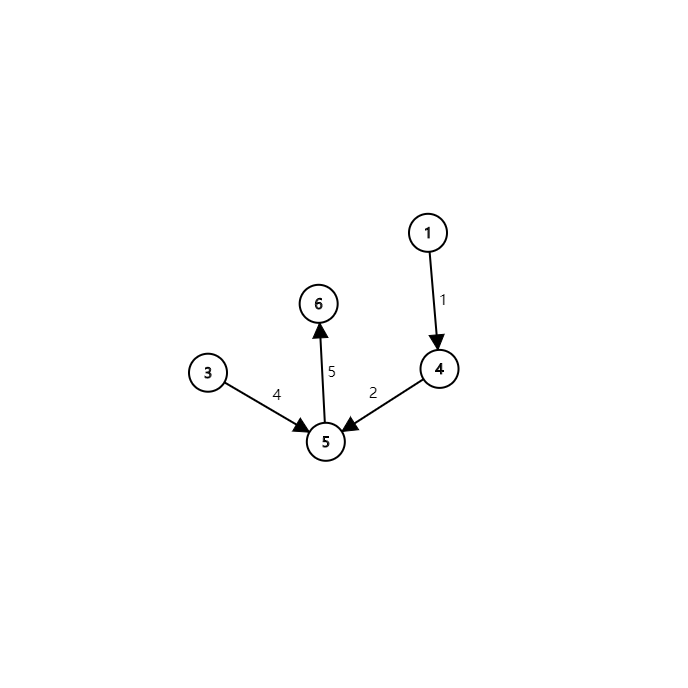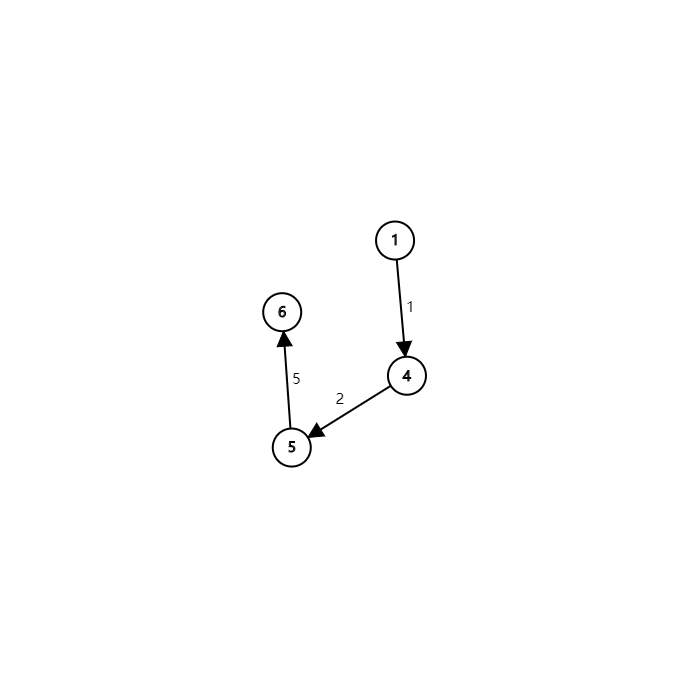# 最长路

## 题目描述

$G$ 为有 $n$ 个顶点的带权有向无环图，$G$ 中各顶点的编号为 $1$$n$，请设计算法，计算图 $G$$<1,n>$ 间的最长路径。

## 输入输出格式

### 输入格式

$2$ 到第 $(m + 1)$ 行，每行 $3$ 个整数 $u, v, w$，代表存在一条从 $u$$v$ 边权为 $w$ 的边。

### 输出格式

$1$$n$ 不联通，请输出 $-1$

## 输入输出样例

### 输入样例 #1

2 1
1 2 1


### 输出样例 #1

1


## 说明

#### 数据规模与约定

• 对于 $20\%$的数据，$n \leq 100$$m \leq 10^3$
• 对于 $40\%$ 的数据，$n \leq 10^3$$m \leq 10^{4}$
• 对于 $100\%$ 的数据，$1 \leq n \leq 1500$$1 \leq m \leq 5 \times 10^4$$1 \leq u, v \leq n$$-10^5 \leq w \leq 10^5$

# 分析

1. 设置 $ind$$dis_n$ 设置为 $-1$$flag_1$ 设置为true。
2. 将所有满足 $ind_i = 0$$i$ push进队列 $q$
3. 若队列非空，取队列第一个作为节点 $u$，并pop掉该节点。
4. 遍历 $u$ 指向的点 $v$，令 $ind_v - 1 \rightarrow ind_v$
5. $flag_u$ 为true，且 $dis_v < dis_u + w$$w$$u \rightarrow v$ 这条边的权值），那么令 $dis_v = dis_u + w$。（最长路核心步骤）
6. 另外，如果 $flag_u$ 为true，那么 $flag_v$ 也要设置为 true。
7. 如果 $ind_v = 0$，将 $v$ 节点入队 $q$
8. 重复 $3 \sim 7$ 步，直到 $q$ 队列为空。此时的 $dis_n$ 即为 $1 \rightarrow n$ 的最长路。

# 代码

/*
* @Author: crab-in-the-northeast
* @Date: 2020-09-25 21:24:06
*/
#include <iostream>
#include <cstdio>
#include <queue>
#include <vector>

const int maxn = 1505;
const int maxm = 50005;

struct edge {
int u, v, dis;
};

int n, m;
std :: vector <edge> G[maxn];
int ind[maxn], dis[maxn];
bool flag[maxn];

void topsort() {
flag = true;
std :: queue <int> q;
for (int i = 1; i <= n; ++i)
if (ind[i] == 0)
q.push(i);

while (!q.empty()) {
int u = q.front();
q.pop();

for (int i = 0; i < G[u].size(); ++i) {
int v = G[u][i].v;
--ind[v];
if (flag[u]) {
if (dis[v] < dis[u] + G[u][i].dis)
dis[v] = dis[u] + G[u][i].dis;
flag[v] = true;
}

if (ind[v] == 0)
q.push(v);
}
}
}

int main() {
std :: scanf("%d %d", &n, &m);
for (int i = 1; i <= m; ++i) {
edge now;
std :: scanf("%d %d %d", &now.u, &now.v, &now.dis);
G[now.u].push_back(now);
++ind[now.v];
}

dis[n] = -1;
topsort();
std :: printf("%d\n", dis[n]);
return 0;
}


# 番外：一个错误的解法# 评测记录

posted @ 2020-09-26 11:38  东北小蟹蟹  阅读(62)  评论(0编辑  收藏